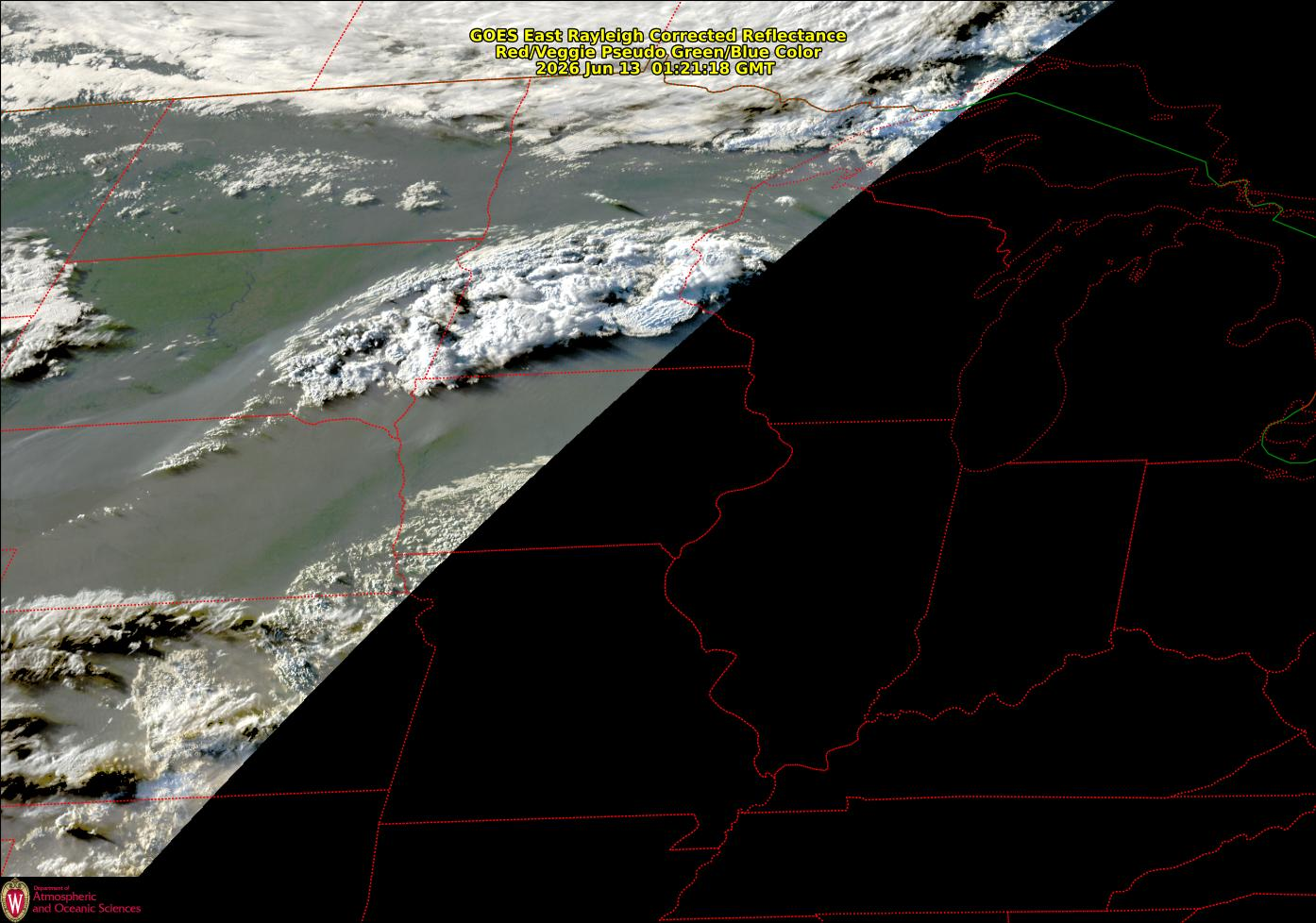2020 Accumulated Growing Degree Days, Williamson county Illinois
 January 31 = 6.5 May 10 = 354 February 29 = 11 May 20 = 471 March 31 = 116 May 30 = 686 April 10 = 187 June 10 = 961 April 20 = 200 June 20 = 1185 April 30 = 274 June 30